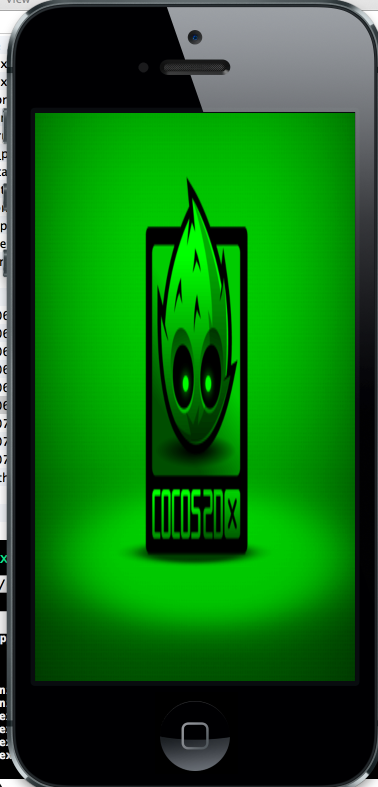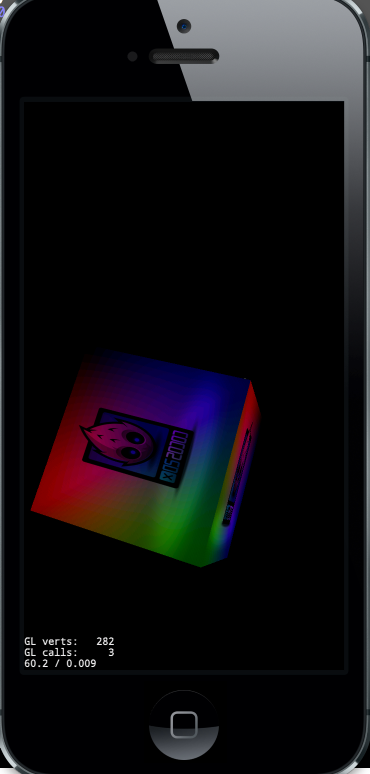## 准备纹理坐标（纹理坐标也叫UV坐标）

 ``````1 2 3 4 5 `````` `````` typedef struct { float Position; float Color; float TexCoord; } Vertex; ``````

 ``````1 2 3 4 5 6 7 `````` `````` Vertex data[] = { { {-1,-1},{0,1,0,1},{0,1}}, { {1,-1},{0,1,0,1},{1,1}}, { {-1,1},{0,1,0,1},{0,0}}, { {1,1},{0,1,0,1},{1,0}} }; ``````

## 生成纹理

 ``````1 `````` `````` GLuint textureId; ``````

 ``````1 `````` `````` glGenTextures(1, &textureId); ``````

## 绑定纹理

 ``````1 2 3 `````` `````` Image *image = new Image; std::string imagePath = FileUtils::getInstance()->fullPathForFilename("HelloWorld.png"); image->initWithImageFile(imagePath); ``````

 ``````1 2 3 4 `````` `````` glTexParameteri(GL_TEXTURE_2D, GL_TEXTURE_MIN_FILTER, GL_LINEAR); glTexParameteri( GL_TEXTURE_2D, GL_TEXTURE_MAG_FILTER, GL_LINEAR ); glTexParameteri( GL_TEXTURE_2D, GL_TEXTURE_WRAP_S, GL_CLAMP_TO_EDGE ); glTexParameteri( GL_TEXTURE_2D, GL_TEXTURE_WRAP_T, GL_CLAMP_TO_EDGE ); ``````

 `````` 1 2 3 4 5 6 7 8 9 10 11 12 13 14 15 `````` ``````unsigned char *imageData = image->getData(); int width = image->getWidth(); int height = image->getHeight(); //调用此方法把imageData的图像数据传递给GPU glTexImage2D(GL_TEXTURE_2D, 0, GL_RGB, width, height, 0, GL_RGB, GL_UNSIGNED_BYTE,//must be GL_UNSIGNED_BYTE imageData); //别忘了释放image内存 CC_SAFE_DELETE(image); ``````

 `````` 1 2 3 4 5 6 7 8 9 10 11 12 13 14 `````` `````` attribute vec2 a_position; attribute vec4 a_color; attribute vec2 a_coord; varying vec4 v_fragmentColor; varying vec2 v_coord; void main() { gl_Position = CC_MVPMatrix * vec4(a_position.xy,0,1); v_fragmentColor = a_color; v_coord = a_coord; } ``````

 `````` 1 2 3 4 5 6 7 8 9 10 `````` `````` varying vec4 v_fragmentColor; varying vec2 v_coord; uniform vec4 u_color; void main() { gl_FragColor = v_fragmentColor * texture2D(CC_Texture0,v_coord); } ``````

## 修改draw call

 ``````1 `````` `````` GL::bindTexture2D(textureId); ``````

## 运行结果## 让立方体不再裸奔

 `````` 1 2 3 4 5 6 7 8 9 10 11 12 13 14 15 16 17 18 19 20 21 22 23 24 25 26 27 28 29 30 31 32 33 `````` ``````#define TEX_COORD_MAX 1 Vertex Vertices[] = { // Front { {1, -1, 0}, {1, 0, 0, 1}, {TEX_COORD_MAX, 0}}, { {1, 1, 0}, {0, 1, 0, 1}, {TEX_COORD_MAX, TEX_COORD_MAX}}, { {-1, 1, 0}, {0, 0, 1, 1}, {0, TEX_COORD_MAX}}, { {-1, -1, 0}, {0, 0, 0, 1}, {0, 0}}, // Back { {1, 1, -2}, {1, 0, 0, 1}, {TEX_COORD_MAX, 0}}, { {-1, -1, -2}, {0, 1, 0, 1}, {TEX_COORD_MAX, TEX_COORD_MAX}}, { {1, -1, -2}, {0, 0, 1, 1}, {0, TEX_COORD_MAX}}, { {-1, 1, -2}, {0, 0, 0, 1}, {0, 0}}, // Left { {-1, -1, 0}, {1, 0, 0, 1}, {TEX_COORD_MAX, 0}}, { {-1, 1, 0}, {0, 1, 0, 1}, {TEX_COORD_MAX, TEX_COORD_MAX}}, { {-1, 1, -2}, {0, 0, 1, 1}, {0, TEX_COORD_MAX}}, { {-1, -1, -2}, {0, 0, 0, 1}, {0, 0}}, // Right { {1, -1, -2}, {1, 0, 0, 1}, {TEX_COORD_MAX, 0}}, { {1, 1, -2}, {0, 1, 0, 1}, {TEX_COORD_MAX, TEX_COORD_MAX}}, { {1, 1, 0}, {0, 0, 1, 1}, {0, TEX_COORD_MAX}}, { {1, -1, 0}, {0, 0, 0, 1}, {0, 0}}, // Top { {1, 1, 0}, {1, 0, 0, 1}, {TEX_COORD_MAX, 0}}, { {1, 1, -2}, {0, 1, 0, 1}, {TEX_COORD_MAX, TEX_COORD_MAX}}, { {-1, 1, -2}, {0, 0, 1, 1}, {0, TEX_COORD_MAX}}, { {-1, 1, 0}, {0, 0, 0, 1}, {0, 0}}, // Bottom { {1, -1, -2}, {1, 0, 0, 1}, {TEX_COORD_MAX, 0}}, { {1, -1, 0}, {0, 1, 0, 1}, {TEX_COORD_MAX, TEX_COORD_MAX}}, { {-1, -1, 0}, {0, 0, 1, 1}, {0, TEX_COORD_MAX}}, { {-1, -1, -2}, {0, 0, 0, 1}, {0, 0}} }; ``````## 结语

 ``````1 2 3 4 5 6 7 `````` `````` //method2: the easier way Texture2D *texture = new Texture2D; texture->initWithImage(image); textureId = texture->getName(); //method3: the easiest way Sprite *sprite = Sprite::create("HelloWorld.png"); textureId = sprite->getTexture()->getName(); ``````

3D旋转立方体(带纹理贴图)源代码下载 master分支 单个图片的纹理贴图源码下载